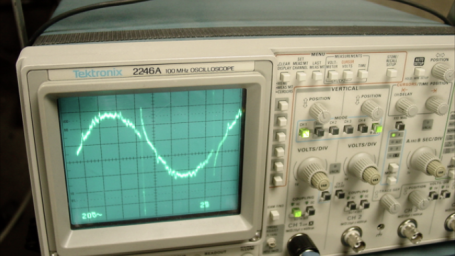Interactive

# AC Power

Martech
Updated Jan 21, 2021

When you finish this lesson, you will be able to calculate for unknown values in a power triangle and calculate power factor. • Describe the trigonometric functions for right triangles • Identify the differences between real, apparent, and reactive power • Describe power factor • Explain power factor correction • Describe power in a purely resistive AC circuit • Describe power in a capacitive or inductive circuit • Apply power value calculation

;